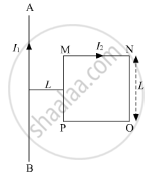# A square shaped current carrying loop MNOP is placed near a straight long current carrying wire AB as shown in the fig. The wire and the loop lie in the same plane. If - Physics

Short Note

A square shaped current carrying loop MNOP is placed near a straight long current carrying wire AB as shown in the fig. The wire and the loop lie in the same plane. If the loop experiences a net force F towards the wire, find the magnitude of the force on the side 'NO' of the loop.#### Solution

The force acting on the section MN and force on section PO will cancel as the wires are located at equal distance from the infinite wire but have current flowing in opposite directions.
The force acting on the whole loop,

"F" = (mu_0I_1I_2L)/(2piL) - (mu_0I_1I_2L)/(2pi(2L)) = (mu_0I_1I_2L)/(4piL)

Towards the wire.
The force acting on the side 'NO' is given by

"F"_"NO" = (mu_0I_1I_2)/(2pi(2L))L = (mu_0I_1I_2)/(4pi) = "F"

Away from the wire.

Is there an error in this question or solution?